# Avoiding Rounding Errors in Floating Point Arithmetic

This post will guide you how to avoid rounding errors in floating point arithmetic in excel. How do I avoid rounding errors in formula results in excel. Many combinations of arithmetic operations on floating point numbers may produce results that appear to be incorrect by very small amounts or rounding errors in cell, how to avoid this error.

## Avoiding Rounding Errors

You can use the Precision as displayed option to avoid rounding errors for floating point arithmetic in excel. Just do the following steps:

#1 go to File tab, click Options, the Excel Options dialog will appear.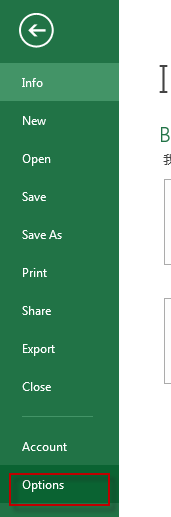#2 click Advanced tab, select the workbook that you want in the When calculating this workbook, check the Set precision as displayed box, and click OK button.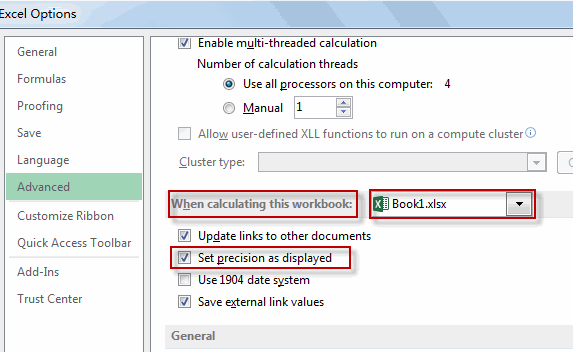#3 right click the cells that you want to format, and click Format Cells from the drop-down menu list. The Format Cells dialog will appear.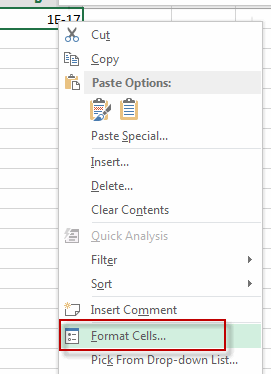#4 switch to Number tab, click Number under Category list, then type a number into the Decimal places box to set the number of decimal places that you want. Click OK button.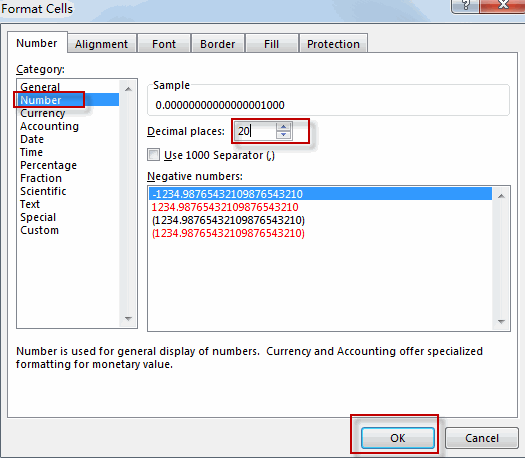#5 let’s see the result.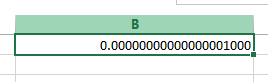Related Posts
If Cell is This Value or That Value

IF function is frequently used in Excel worksheet to return you expect “true value” or “false value” based on the result of logical test. If you want to see if a cell is A or B, and if one of ...

If Value is Greater Than A Certain Value

IF function is frequently used in Excel worksheet to return you expect “true value” or “false value” based on the logical test result. If you want to see if a value in one cell is greater than a specific value, ...

If Cell is Not Blank

IF function is frequently used in Excel worksheet to return you expect “true value” or “false value” based on the result of created logical test. If you want to see if a cell is blank or not, and leave some ...

VBA Macro For VLOOKUP From Another Sheet

In the previous post, you should know that how to fix or remove the #N/A error when using VLOOKUP formula to lookup value from another sheet. And this post will show you how to use VBA code to vlookup data ...

If Cell is Blank

IF function is frequently used in Excel worksheet to return you expect “true value” or “false value” based on the result of created logical test. If you want to see if a cell is blank or not, and leave some ...

If Cell Equals Certain Text String

IF function is frequently used in Excel worksheet to return you expect “true value” or “false value” based on the result of created logical test. If you want to see if cell equals a certain text string like “Win”, you ...

If Cell Contains Either Text1 or Text2

IF function is frequently used in Excel worksheet to return “true value” or “false value” based on the logical test result. If you want to see if cell contains certain substring1 like “abc” or substring2 like “def”, and returns true ...

If Cell Contains Certain Text OR Equals Certain Text

IF cell equals certain text IF function is frequently used in Excel worksheet to return “true value” or “false value” based on the logical test result. If you want to test values to see if they equal certain text like ...

VLOOKUP From Another Sheet Not Working

In the previous post, you should know that how to fix or remove the #N/A error when using VLOOKUP formula to lookup value from another sheet. And this post will show you reasons why your VLOOKUP formula is not working ...

If Cell Begins with One of Three Supplied Characters

If you want to test values to see if they begin with some given specific characters like “x”, ”y”, or “z”, you can create a formula with COUNTIF and SUM functions to return results. EXAMPLE You can see “TRUE” or ...

Sidebar